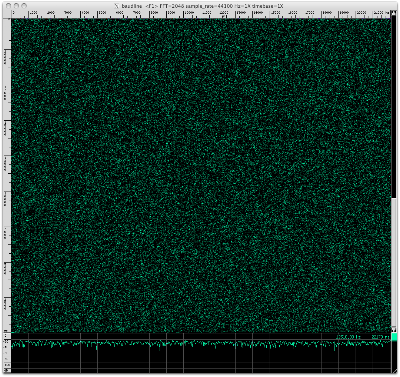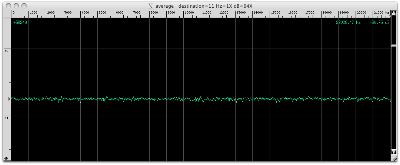## Tuesday, November 09, 2010

### Carl Sagan's birthday pi

The NASA Kepler mission and the SETI Institute are celebrating Carl Sagan's birthday with an essay contest. I thought that I'd do something different and honor Carl Sagan by analyzing the digits of pi with the baudline signal analyzer. In Carl Sagan's novel "Contact" finding a signal hidden in the digits of pi was a theme element that was removed from the movie version. Since I've been spending a lot of my free time analyzing setiQuest data sets it seems rational to apply some of those same techniques to the irrational number pi.

So my plan is to use baudline and conduct Fourier analysis on the digits of pi in binary (base-2). Here is a description of the procedure:
1. Compute millions of decimal digits of pi.
2. Convert the decimal digits of pi to binary (base-2).
3. Analyze the binary digits with the baudline signal analyzer.

Existing software available on the Internet was used to calculate about 50 million decimal digits of pi. I wrote a simple O(n^2) base conversion routine that did multiple-digit base multiply and carry using integer division and modulo. For base-2 conversion accuracy verification I used the amazing Bailey–Borwein–Plouffe (BBP) formula algorithm to spot check several hexadecimal digits. The data was then feed into baudline using the standard input (stdin) or the raw parameter interface.

white uniform noise
The digits of pi are believed to be normal in that their distribution is random. Before looking at pi let us first take a look at the spectral characteristics of white uniform noise. Baudline settings:
1. Tone Generator set to output white uniform noise.
2. Input Devices tone generator loopback enabled.
3. Input Channel Mapping operation set to clip.
Here is a spectrogram of about one million samples of clipped uniform white noise:Here is an Average spectrum of 67 Msamples:pi binary (base-2)
The decimal (base-10) digits of pi were converted to binary (base-2). Here is a picture of the binary waveform:Here is a spectrogram of about one million samples of binary (base-2) pi:Here is an Average spectrum of 67 Msamples:Comparison
Let us compare the above uniform white noise and binary pi spectral displays. Click on the two spectrograms above for full size versions and see if you can find any significant differences. They look very similar to me. The two Average spectrums are fairly flat with equal energy and variance. Nothing stands out as odd, unusual, or different. So the conclusion from the perspective of these basic frequency domain tests is that white uniform noise is indistinguishable from the binary digits of pi.

If there is some hidden structure in pi then more sophisticated DSP techniques will need to be developed and utilized. Stay tuned ...